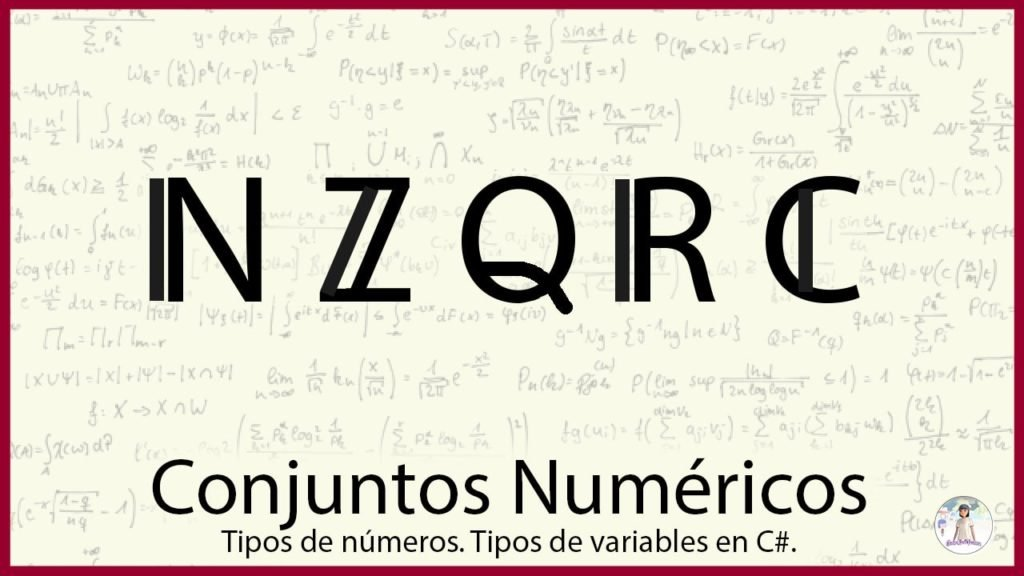# Numerical sets. Types of numbers and application

## Introduction

Numeric sets are used to group numbers that have similar characteristics. It is one of the basic concepts of mathematics so it is important to understand what they are and what characteristics each has.

## What are number sets used for?

Numeric sets are used to separate numbers into different classes that have similar properties.

We must see this simply as a form of organization, in which given any number we say that this number belongs to such a set.

## Numerical Sets

The basic numerical sets are as follows:

• Natural – ℕ
• Integer – ℤ
• Rational – ℚ
• Real – ℝ
• Complex – ℂ

Each more general set encompasses the previous set, i.e. for example all natural numbers are integers, but not all integers are natural.

### Set of Natural Numbers (ℕ)

This set is made up of the numbers {1,2,3,…} (the suspension points indicate that the enumeration continues indefinitely), these numbers are all positive and represent integer magnitudes, i.e. they have no decimal part.

### Set of integer numbers (ℤ)

If to the natural numbers we add the number 0 and the negative numbers without decimal part we obtain the set of integers. {…,-3,-2,-1,0,1,2,3,…}.

With negative numbers we can represent subtraction operations, missing magnitudes, values below the reference zero and so on.

Some examples are outgoing flows of money, that is, money that we pay and subtract from what we have; temperatures below zero are expressed as negative values of degrees Celsius.

### Sets of Rational Numbers (ℚ)

The set of rational numbers arises from making divisions of two whole numbers. For example 1 divided 2 is an operation that results in a number that is smaller than 1 but larger than 0.

These numbers are used to represent non integer magnitudes, for example variables of continuous nature such as speed, weight, electric current; to express fractional quantities such as half a kilo of flour are 0.5 kg of flour.

### Set of Real Numbers (ℝ)

If to the set of rational numbers we add the set of irrational numbers we get the set of real numbers.

Irrational numbers arise from performing certain operations and it is not possible to express them as the quotient between two whole numbers.

An example of this type of numbers is the well-known Pi number which is composed of infinite decimal digits.

### Set of Complex Numbers (ℂ)

If we add imaginary numbers to the set of real numbers, we get the set of complex numbers. Any number we choose will be, in general terms, a complex number.

The imaginary number i is the result of the square root of -1.

Complex numbers are composed of a real part and an imaginary part and are very useful for the study of electrical circuits involving capacitive or inductive elements. They are also used in Fourier transform calculations.

## Numerical Sets in Videogames

### Modeling of game elements

To model the behavior of different elements in our game we will probably need to use different types of numbers.

For example a character may have a standard of living represented by hearts. When it is damaged it loses a heart and when it takes a healing potion it recovers a heart. In this case the health level is a whole magnitude.

We can also think, for example, that each heart can be divided into four parts and each blow received takes away a quarter of our heart. In addition it could be that a strong enemy takes away three quarters of our heart with each blow. In this case we are thinking of hearts as rational magnitudes and we are going to need decimal numbers to represent them.

It all depends on how we want our model to be.

### Numerical sets in programming

To represent the different types of numbers we need to build models, we have elements called variables, which are spaces in the memory where information is stored.

### Types of numerical variables in C#

The programming language we use in Unity offers us different types of variables.

#### Integers

To represent integer magnitudes we have for example the byte, the short, int and long

Each has its own range of representation, for example the int allows us to represent integers that are between -2,147,483,648 and 2,147,483,647. The short instead allows us to represent numbers between -32,768 and 32,767, which is also a fairly large range.

By default we are going to use the int variable type to represent integer magnitudes.

#### Reals

The floating point system is used to represent decimal values in computation.

We have 32-bit float type variables, 64-bit double type variables and 128-bit decimal type variables. Each one has a certain range of representation and precision.

In computation, infinite digits cannot be stored, so irrational numbers will be represented as rational numbers and a truncation error will arise.

#### Complex

Complex numbers can be represented with two real numbers, one is the real part and the other is the imaginary part, the latter will be multiplied by the number i. As expressed in the following equation:

Z = X + i . Y

X and Y being real numbers and Z being a complex number.

We can represent complex numbers using two floats with significant names for the real and imaginary parts, we can use two-component vectors, or we can use the Complex class of C#.

## Conclusion

Numeric sets are names we use to classify the different types of numbers that exist.

Knowing the different types of numbers and their properties will help us in building models for our games.

Para implementar los números en programación contamos con elementos llamados variables. Existen distintos tipos de variables para representar números enteros y racionales, cada tipo tiene su propio rango de representación.